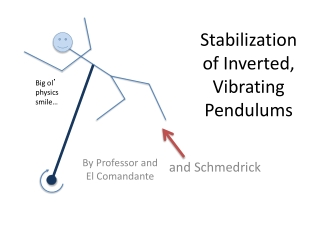DownloadDownload PresentationStabilization of Inverted, Vibrating Pendulums

# Stabilization of Inverted, Vibrating Pendulums

Download Presentation## Stabilization of Inverted, Vibrating Pendulums

- - - - - - - - - - - - - - - - - - - - - - - - - - - E N D - - - - - - - - - - - - - - - - - - - - - - - - - - -
##### Presentation Transcript

1. Stabilization of Inverted, Vibrating Pendulums Big ol’ physics smile… By Professor and El Comandante and Schmedrick

2. EquilibriumNecessarily: the sums of forces and torques acting on an object in equilibrium are each zero • Stable Equilibrium—E is constant,and original U is minimum, small displacement results in return to original position . • Neutral Equilibrium—U is constant at all times. Displacement causes system to remain in that state . • Unstable Equilibrium—Original U is maximum, E technically has no upper bound. • Static Equilibrium—the center of mass is at rest while in any kind of equilibrium. • Dynamic Equilibrium—(translational or rotational) the center of mass is moving at a constant velocity. ω = constant

3. Simple Pendulum Review Schmedrick says: The restoring torque for a simple rigid pendulum displaced by a small angle is MgrsinΘ ≈ mgrΘ and that τ = Ια… MgrΘ = Ια  grΘ = r2Θ’’ α = -gsinΘ⁄r α ≈ g ⁄ r Where g is the only force-provider The pendulum is not in equilibrium until it is at rest in the vertical position: stable, static equilibrium. r Θ m mgcosΘ mgsinΘ mg

4. Mechanical Design Rigid pendulum • Oscillations exert external force: • Downward force when pivot experiences h’’(t) < 0 ; help gravity. • Upward when h’’(t) > 0 ; opposes gravity. • Zero force only when h’’(t) = 0 (momentarily, g is only force-provider) Pivot height as a function of time h(t) = Acos(ωt) 1 pivot ω shaft Differentiating: h’(t) = -Aωsin(ωt) h’’(t) = -Aω2cos(ωt) = translational acceleration due to motor 2 A pivot Disk load Motor face

5. Analysis of Motion m mgsinΘ • h’’(t) is sinusoidal and >> g, so Fnet ≈ 0 over long times • Torque due to gravity tends to flip the pendulum down, however, limt ∞ (τnet)≠0 , we will see why… • Also, initial angle of deflection given; friction in joints and air resistance are present. Imperfections in ω of motor. mgcosΘ mg Θ r g h’’(t) = -Aω2cos(ωt)

6. Torque Due to Vibration: 1 Full Period 1 1 1 Θ3 Note: + angular accelerations are toward vertical, + translational accelerations are up Θ1 #1 Pivot accelerates down towards midpoint, force applied over r*sinΘ1; result: Θ  Not very large increase in Θ b/ small torque, stabilized h’’(t) > 0 h’’(t) 2 2 2 Large Torque (about mass at end of pendulum arm) #3 On the way from 2 to 1, the angle opens, but there is less α to open it, so by the time the pivot is at 1, Θ3 < Θ1 Therefore, with each period, the angle at 1 decreases, causing stabilization. #2 Same |h’’(t)|, however, a smaller τ is applied b/c Θ2 < Θ1. Therefore, the pendulum experiences less α away from the vertical than it did toward the vertical in case #1 Θ2 h’’(t) Small Torque

7. Explanation of Stability • Gravity can be ignored when ωmotor is great enough to cause large vertical accelerations • Downward linear accelerations matter more because they operate on larger moment arms (in general) • …causing the average τ of “angle-closing” inertial forces to overcome “angle-opening” inertial forces (and g) over the long run. • Conclusion: “with gravity, the inverted pendulum is stable wrt small deviations from vertical…”.

8. Mathieu’s Equation: α(t) α due to gravity is in competition with oscillatory accelerations due to the pivot and motor. g is always present, but with the motor: Differentiating: h(t) = Acos(ωt) h’(t) = -Aωsin(ωt) h’’(t) = -Aω2cos(ωt) = translational acceleration due to motor 1) Linear acceleration at any time: 2) Substitute a(t) into the “usual” angular acceleration eqn: . But assuming that “g” is a(t) from (1) since “gravity” has become more complicated due to artificial gravity of the motor… 

9. Conditions for Stability From ; (ω0)2 = g/r • Mathieu’s equation yields stable values for: • α < 0 when |β| = .450 (where β =√2α  

10. Works Cited • Acheson, D. J. From Calculus to Chaos: An Introduction to Dynamics. Oxford: Oxford UP, 1997. Print. Acheson, D. J. • "A Pendulum Theorem." The British Royal Society (1993): 239-45. Print. Butikov, Eugene I. • "On the Dynamic Stabilization of an Inverted Pendulum." American Journal of Physics 69.7 (2001): 755-68. Print. French, A. P. • Newtonian Mechanics. New York: W. W. Norton & Co, 1965. Print. The MIT Introductory Physics Ser. Hibbeler, R. C. • Engineering Mechanics. New York: Macmillan, 1986. Print.